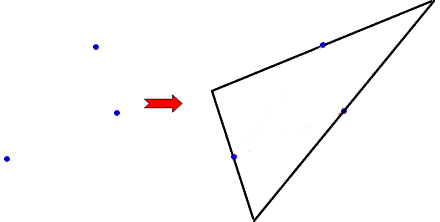#### You may also like### Fitting In

The largest square which fits into a circle is ABCD and EFGH is a square with G and H on the line CD and E and F on the circumference of the circle. Show that AB = 5EF. Similarly the largest equilateral triangle which fits into a circle is LMN and PQR is an equilateral triangle with P and Q on the line LM and R on the circumference of the circle. Show that LM = 3PQ### Look Before You Leap

Can you spot a cunning way to work out the missing length?Two ladders are propped up against facing walls. The end of the first ladder is 10 metres above the foot of the first wall. The end of the second ladder is 5 metres above the foot of the second wall. At what height do the ladders cross?

# Triangle Midpoints

##### Age 14 to 16Challenge Level

Given the three midpoints of the sides of a triangle, can you find a way to construct the original triangle?

For example:Choose any three points.
Can you construct a triangle such that your three points are the midpoints of its sides?

Is there more than one possible triangle for any given set of midpoints?